BLS （Boneh-Lynn-Shacham）签名算法是一种可以实现签名聚合和密钥聚合的算法，它可以把一笔交易中的所有签名和公钥合并成单个签名和公钥，且合并过程不可见（无从追溯这个签名或公钥是否通过合并而来）。

## 1.基本原理

e(P, Q) → n
e(x×P, Q) = e(P, x×Q)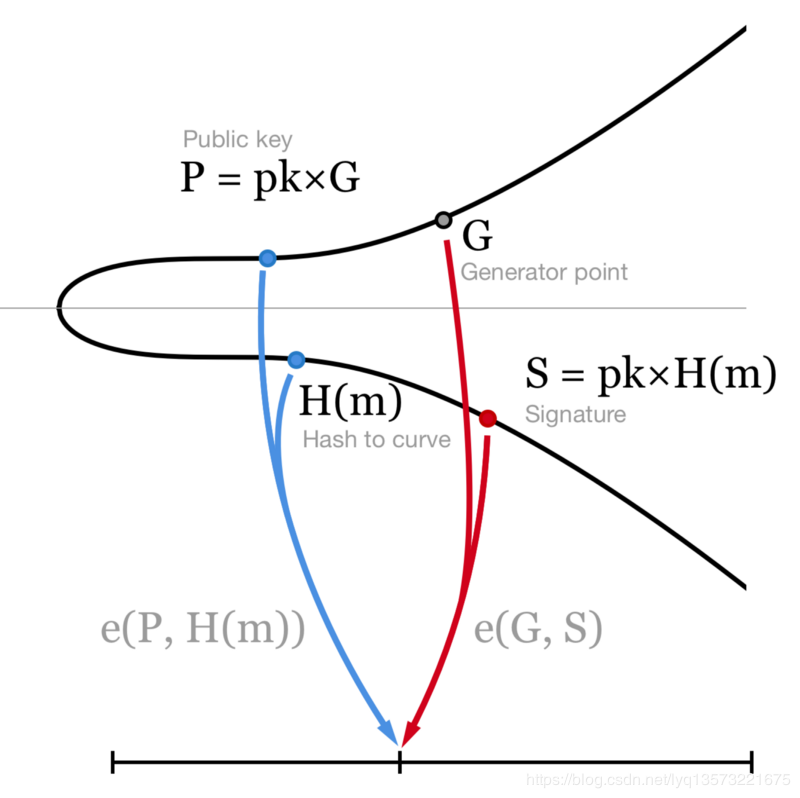## 2.主要流程

2.1 每个节点生成一组私钥$r_0, r_1, …, r_{m-1} r = a, b, c, d, …$这些私钥是一个m阶多项式的参数: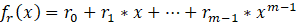2.2 每个节点对其他节点x=1,2,…,n生成多个多项式的值， 最简单的情况就是用其他节点的编号。生成后在私底下秘密传递给相对应的节点（一般可以通过用对方的非对称公钥加密后发送）：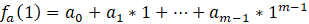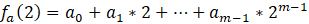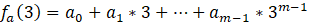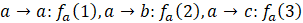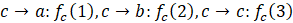2.3 每个节点公开公钥信息：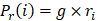2.4 每个节点可以验证收到的 $f_r(x)$ 是否正确，以a为例，验证方法为：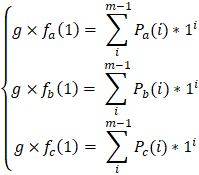2.5 如果验证有错，广播错误，这里会涉及一些反馈以及contest的过程。

2.6 如果所有的节点的验证都没有错误，每个节点达成一致，每个节点本地获得的信息如下表：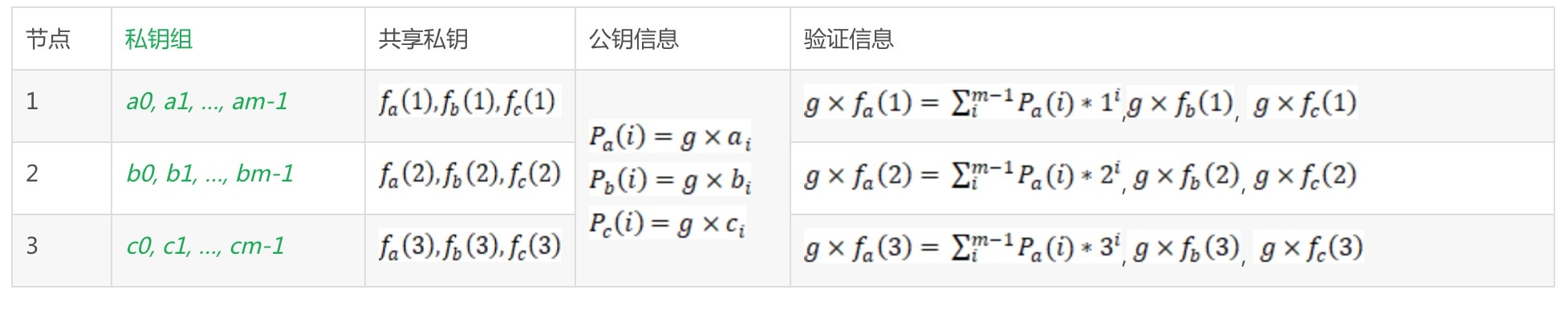2.7 这样，系统中的全局私钥为$(a_0 + b_0 + c_0)$，全局公钥为$g × (a_0 + b_0 + c_0)$。但是没有一个人能够知道全局私钥。如果知道了m个共享私钥片段$f_r(1…m)$，就可以计算出全局私钥。

## 3.应用示例

1. 对于一个信息s，先计算出其hash在曲线上的映射$H(s)$，参见文章开头的插图
2. 每个节点公布$H_r(s) = \sum f_r(i) × H(s)$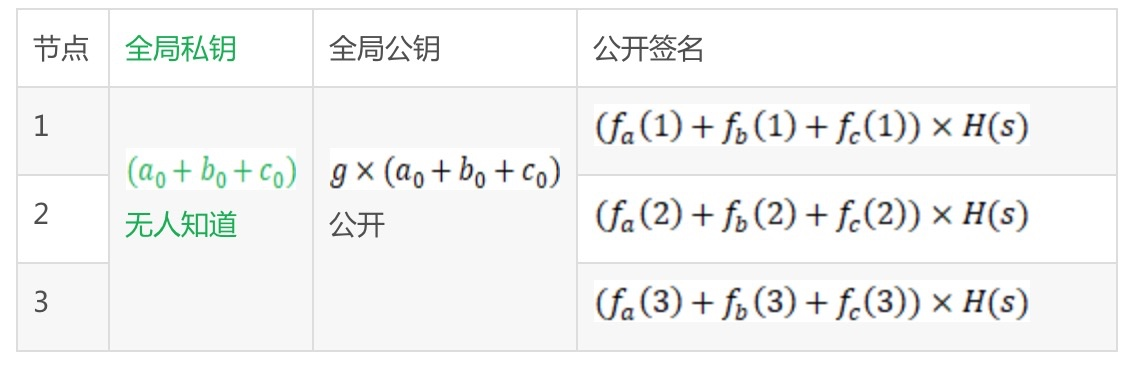3. 这样任意m个$H_r(s)$可以计算出$(a_0 + b_0 + c_0) × H(s)$，这个就是信息s的全局签名。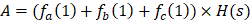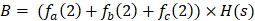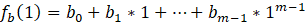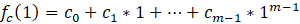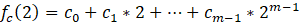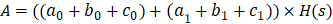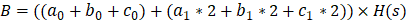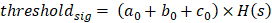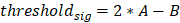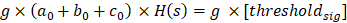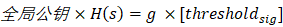0%